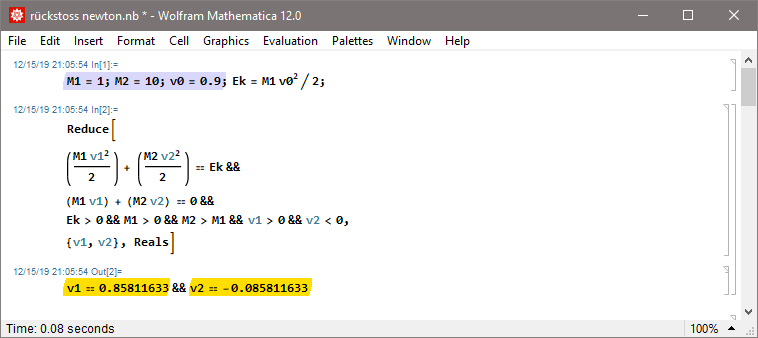## Relativistic Recoil

English Version
Yukterez
Beiträge: 269
Registriert: Mi 21. Okt 2015, 02:16

### Relativistic RecoilThis is the english version.Deutschsprachige Version: hier entlangno GravityExercise: A projectile has a velocity of v0=0.9 (in natural units of c) when shot from a clamped in canon. If the canon is not clamped in but floating freely, and the mass of the canon is 10× that of the projectile, what would the velocity v1 of the projectile be, and how high the recoil velocity v2 of the canon?1) Newton2) EinsteinSimon Tyran @ minds || gab || parler || wikipedia || stackexchange || wolframYukterez
Beiträge: 269
Registriert: Mi 21. Okt 2015, 02:16

### Relativistic Recoilwith GravityExercise: A satellite orbits around a black hole's ISCO. How much energy is required to launch a projectile with the required escape velocity to escape to infinity if the mass of the satellite is 10× higher than the mass of the projectile?

Calculation: vPro: prograde velocity of the satellite, vEsc: escape velocity (both measured in the frame of a locally stationary and angular momentum free observer), vDif: required projectile velocity, vRec: recoil (both in the satellite's frame of reference), Ek: required energy to launch the projectile (in natural units of mc², where m is the mass of the projectile).

Animation: on the left the projectile (red) is launched with the required escape velocity, and the satellite's orbital velocity is reduced by the recoil. On the right a second projectile (orange) is launched into the opposite direction to cancel out the recoil so the satellite can keep a constant velocity. Before the shot at t=0 the numerical display shows the orbital parameters of the satellite, and after t=0 those of the projectile.1) KeplerSimulation (click on the image to lower the frame rate):Code: .txt, worksheet: .nb2) SchwarzschildSimulation (click on the image to lower the frame rate):Code: .txt, worksheet: .nb3) KerrSimulation (click on the image to lower the frame rate):Code: .txt, worksheet: .nbSimon Tyran @ minds || gab || parler || wikipedia || stackexchange || wolfram